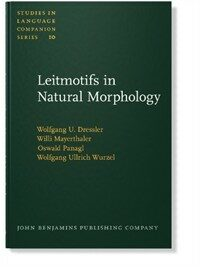> 상세정보

# 상세정보## Leitmotifs in natural morphology (1회 대출)

자료유형
단행본
개인저자
Dressler, Wolfgang U, 1939-.
서명 / 저자사항
Leitmotifs in natural morphology / by Wolfgang U. Dressler ... [et al.] ; edited by Wolgang U. Dressler.
발행사항
Amsterdam ;   Philadelphia :   J. Benjamins Pub. Co.,   1987.
형태사항
viii, 168 p. : ill. ; 23 cm.
총서사항
Studies in language companion series,0165-7763 ; v. 10
ISBN
9027230099 (alk. paper) :
서지주기
Includes bibliographical references (p. -164) and index.
일반주제명
Grammar, Comparative and general -- Morphology. Naturalness (Linguistics)
 000 01081camuu2200277 a 4500 001 000045268331 005 20060616142602 008 870721s1987 ne a b 001 0 eng 010 ▼a 87021791 020 ▼a 9027230099 (alk. paper) : ▼c \$26.00 035 ▼a (KERIS)BIB000000247030 040 ▼a 211032 ▼c 211032 ▼d 211009 050 0 0 ▼a P241 ▼b .L45 1987 082 0 0 ▼a 415 ▼2 20 090 ▼a 415 ▼b L533 245 0 0 ▼a Leitmotifs in natural morphology / ▼c by Wolfgang U. Dressler ... [et al.] ; edited by Wolgang U. Dressler. 260 ▼a Amsterdam ; ▼a Philadelphia : ▼b J. Benjamins Pub. Co., ▼c 1987. 300 ▼a viii, 168 p. : ▼b ill. ; ▼c 23 cm. 440 0 ▼a Studies in language companion series, ▼x 0165-7763 ; ▼v v. 10 504 ▼a Includes bibliographical references (p. -164) and index. 650 0 ▼a Grammar, Comparative and general ▼x Morphology. 650 0 ▼a Naturalness (Linguistics) 700 1 ▼a Dressler, Wolfgang U, ▼d 1939-. 945 ▼a KINS

### 소장정보

No. 소장처 청구기호 등록번호 도서상태 반납예정일 예약 서비스
No. 1 소장처 청구기호 415 L533 등록번호 111366744 도서상태 대출가능 반납예정일 예약 서비스

### 컨텐츠정보

#### 목차

```
CONTENTS
Preface
A. INTRODUCTION
I. Introduction = 3
1. What is naturalness? = 3
2. Inflection and word formation = 4
3. Towards a theory of morphological naturalness = 7
4. The structure of a theory of naturalness = 8
5. Evidence for naturalness = 12
6. The semiotic framework of natural morphology = 15
7. Divergences and convergences among proponents of natural approaches to morphology = 18
Notes = 20
B. NATURALNESS IN INFLECTION
I. System-independent morphological naturalness / Willi Mayerthaler = 25
0. Introduction = 25
1. Universal grammar and markedness = 31
2. Preference theories = 33
2.1. Preferences, open systems, and some misunderstandings = 34
2.2. On the relation of preference theories and the theory of grammar = 38
2.3. Foundations of markedness relations = 39
3. Some other prerequisites of theoretical morphology = 42
3.1. Some remarks about the lexicon = 44
3.1.1. Which word classes are supposed to be universal? = 46
4. Principles of morphological markedness theory = 48
4.1. Summary of symbolic generalizations = 50
5. What is a 'theory'? = 52
5.1. Shortcomings of system-independent naturalness theory = 53
6. Appendix on attractors in catastrophe theory = 55
Notes = 57
II. System-dependent morphological naturalness in inflection / Wolfgang U. Wurzel = 59
0. Naturalness, morphology and language system = 59
1. System-dependent naturalness I: System-congruity = 62
1.1. System-defining structural properties and system-congruity = 62
1.2. System-defining structural properties and morphological change = 65
1.3. System-congruity and system-independent naturalness = 69
1.4. Example : Noun inflection in New High German = 71
2. System-dependent naturalness II : Stability of inflectional classes = 76
2.1. Structure of inflectional paradigms : Implicative paradigm structure conditions = 76
2.2. Dominant paradigm structure conditions, stable and unstable inflectional classes = 78
2.3. Superstable markers = 82
2.4. Change in the stability of inflectional classes = 83
2.5. Stability of inflectional classes and system-independent naturalness = 86
3. System-congruity and class-stability: Productivity in inflectional morphology = 87
3.1. Primary productivity = 87
3.2. Secondary productivity = 90
4. Naturalness principles of inflectional morphology = 92
Notes = 94
C. NATURALNESS IN WORD FORMATION
I. Word formation as part of natural morphology / Wolfgang U. Dressier = 99
1. Introduction = 99
2. Iconicity in word formation = 101
2.1. Images and diagrams = 101
2.2. Diagrammaticity of compounds = 101
2.3. Constructional iconicity = 102
2.4. Morphotactic transparency = 102
2.5. Conversion and suppletion = 103
2.6. Subtraction = 104
2.7. Scales of naturalness = 104
2.8. Predictions = 105
2.9. Typological prospect = 110
3. Indexicality in word formation = 110
4. The scale of (bi)uniqueness in word formation = 111
5. Signans shapes adequate for motivation in word formation = 116
6. Typological adequacy of word formation rules = 118
7. System adequacy (congruity) of word formation rules = 121
8. Inflection vs. derivation again = 123
Notes = 124
II. Productivity and diachronic change in morphology / Oswald PanagI = 127
0. Introduction = 127
1. Interpretations of productivity = 128
1.1. Quantitative criterion = 131
1.2. Qualitative criterion = 131
1.3. Grammatical criterion = 131
1.4. Typological criterion = 131
2. Vedic root-nouns and Ancient Greek diminutives = 132
3. Semantic predictability (transparency) = 133
4. Transition from word formation into inflection = 134
5. Hierarchy of productivity in types of word formation = 136
6. Conclusion on productivity in corpus languages = 137
The Latino Supine in -(t)um - a Case Study of Categorial Change
7. From derivational to inflectional morphology = 137
8. Reasons for loss of productivity = 143
9. Indo-European cognates = 149
Notes = 150
Abbreviations = 153
General bibliography = 155
Subject index = 165

```

### 관련분야 신착자료

#### Grammaticalization / 1st ed

Narrog, Heiko (2021)